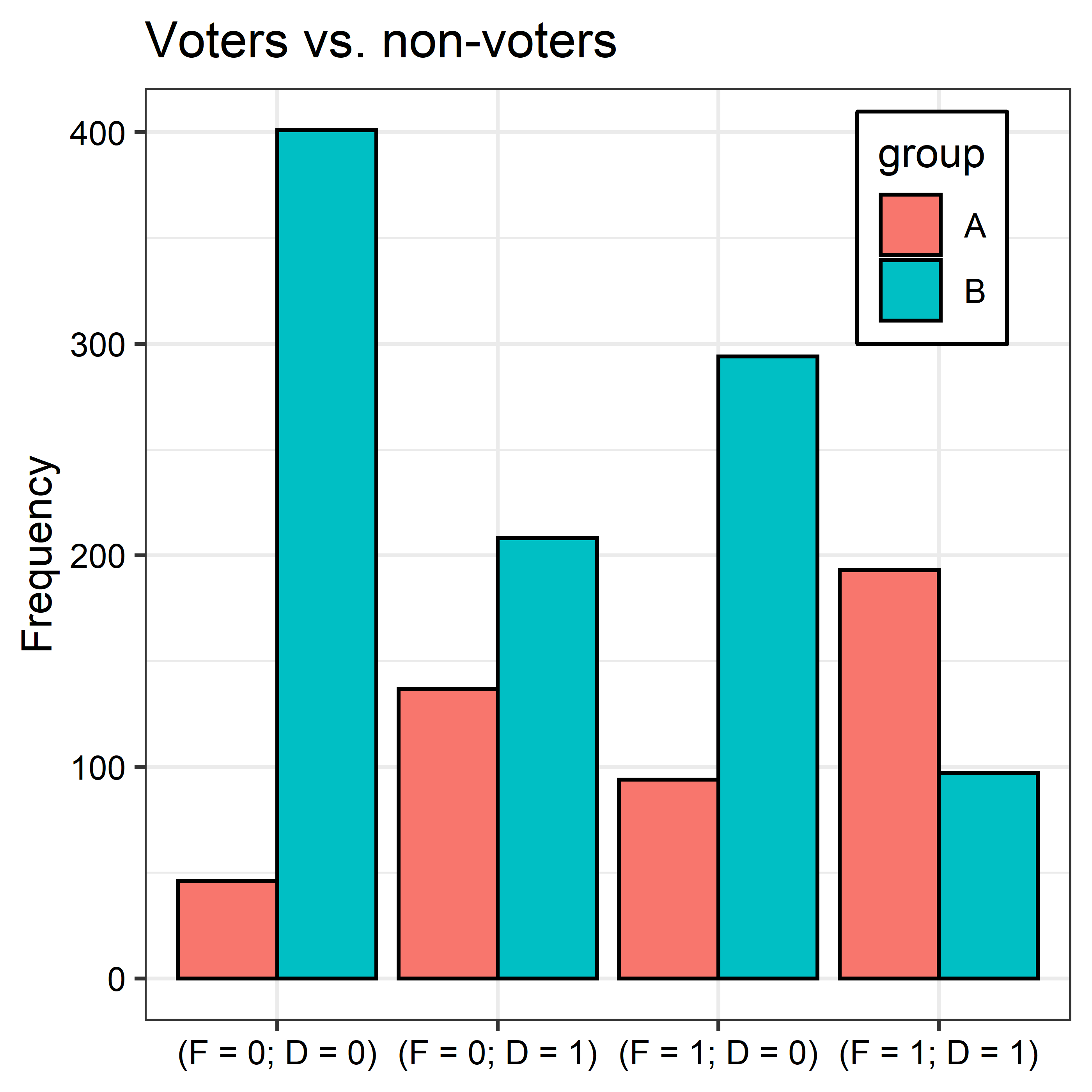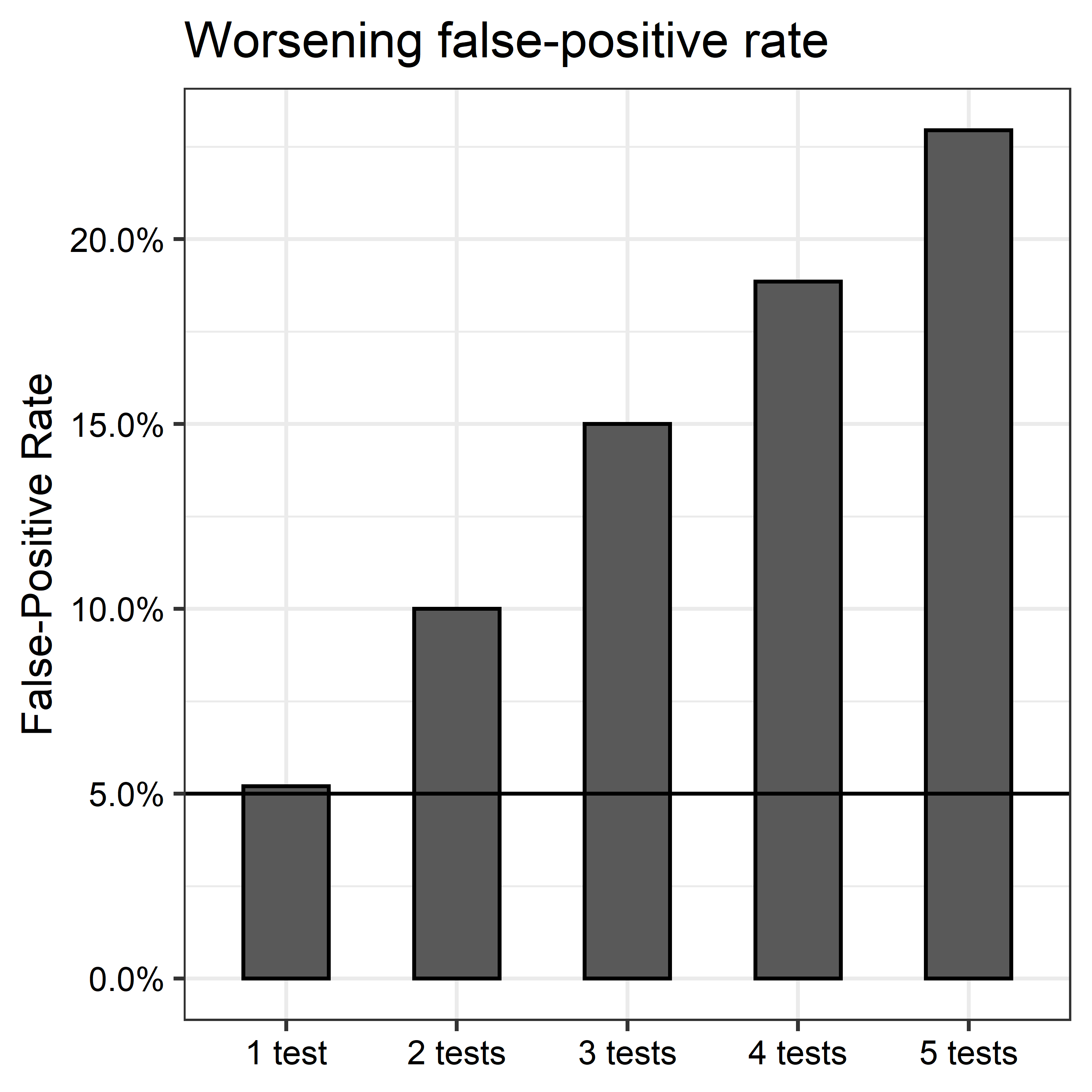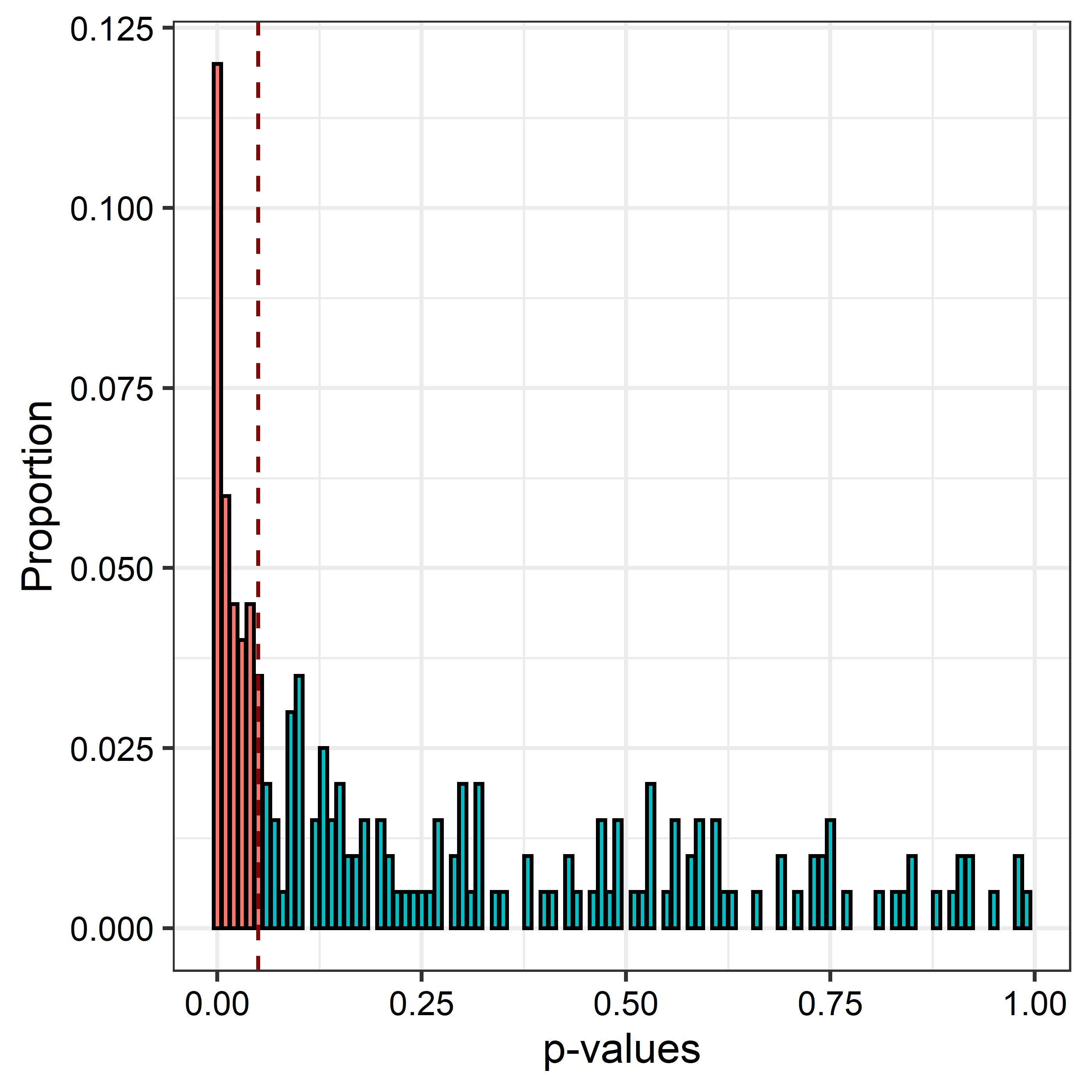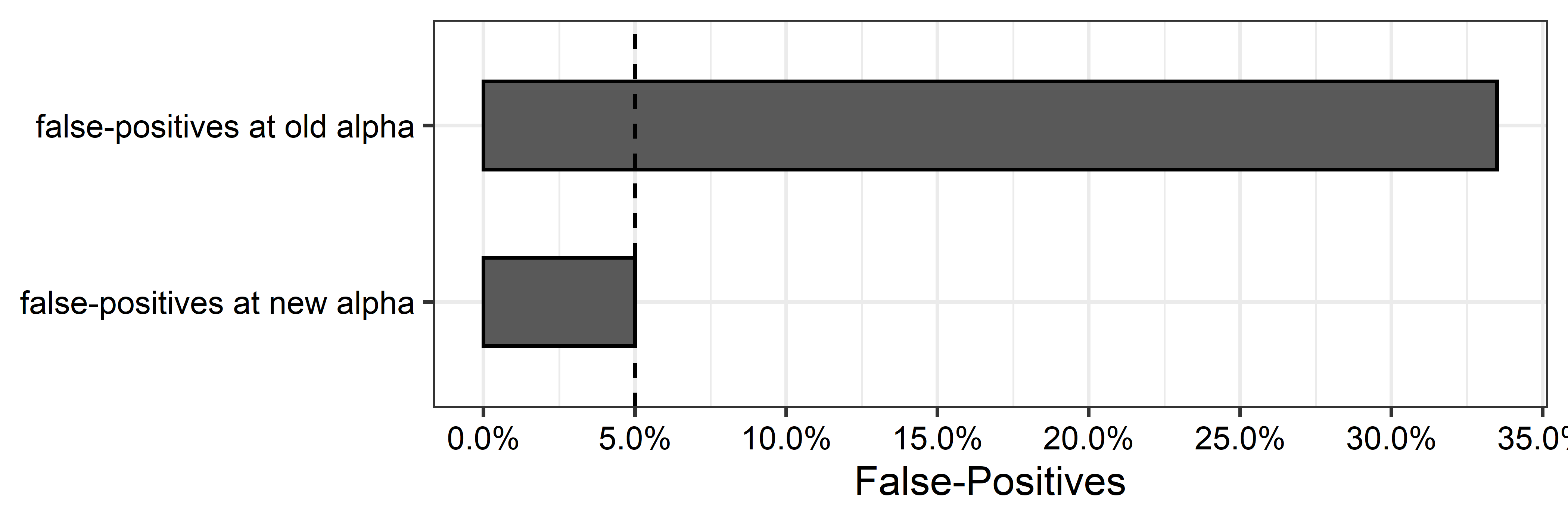Back to Blog

There’s a multiple comparisons problem with multinomial tests, and it has a simple solution. In this post, I demonstrate what the problem is and how to overcome it.

## Multinomial Tests

Multinomial tests help in comparing discrete distributions. For example, one might use a multinomial test to determine whether two subpopulations look the same with respect to demographic characteristics. Supose we have a dataset with individuals divided into two groups. In group A we have individuals who voted in a US mid-term election. In group B we have those who did not. For simplicity’s sake, assume the only demographic variables we have for individuals in each group are gender (F = 1 if female, 0 if male) and whether an individual has at least a four-year degree (D = 1 if yes, 0 otherwise).

For each of the groups, there are four unique strata into which individuals can fall:

1. Female and a four-year degree (F = 1 and D = 1);
2. Female and no degree (F = 1 and D = 0);
3. Male and a four-year degree (F = 0 and D = 1);
4. Male and no degree (F = 1 and D = 0).

The frequency distributions of each strata differ between groups. As the below figure summarizes, women and those with a four-year degree are over-represented among voters (group A) and are under-represented among non-voters (group B).The groups clearly look different, but is this difference statistically detectable? We can answer this question using a multinomial test, such as chi-squared:

library(tidyverse) # for grammar
library(seerrr)    # for simulations
library(XNomial)   # for multinomial tests

# perform chi-squared test
test <- xmonte(
obs = dt$freq[dt$group == "A"],
exp = dt$freq[dt$group == "B"],
statName = "Chisq"
)

##
## P value (Chisq) = 0 ± 0

test[c("observedChi", "pChi")] %>% bind_cols()

## # A tibble: 1 x 2
##   observedChi  pChi
##         <dbl> <dbl>
## 1        644.     0


The output shows the computed chi-squared statistic with its Monte Carlo simulated p-value (this is a more robust alternative to a test based on the asymtotic chi-squared distribution). The p-value is well below the usual 0.05 threshhold, meaning we can reject the null that the subpulations are drawn from the same multinomial distribution.

## The Problem

The convenience of a multinomial test in this context is its relatively few assumptions. It simply considers whether the frequencies in each strata between groups A and B are too different from one another to be the result of chance. Beyond this, it makes no assumptions about the form or direction of this difference. This is a good justification for using this kind of test for addressing questions like that posed above.

However, an often overlooked limitation of this approach is that multinomial tests, though not explicitly specified as such, are based on multiple comparisons. Unlike a t-test, for example, which considers the likelihood that the difference in means of two groups is greater than we’d expect by chance, a multinomial test like chi-squared considers differences across multiple strata between groups. In the case of the above example, four comparisons are being made on the basis of voting status—not just one.

This is problematic from a testing perspective because multiple comparisons are subject to what’s known as the multiple testing problem. In short, as the number of statistical tests being considered grows, the likelihood of falsely rejecting the null hypothesis increases. This is what’s called the type I error or false positive rate.

That multinomial tests are subject to this problem isn’t obvious at first blush because they involve the estimation of a single statistic to summarize the differences between groups, and this statistic has one unique p-value. But the problem exists nonetheless. The below simulation illustrates this point.

When the null hypothesis is true, we expect to get a false positive 5 percent of the time. In the below code a possible distribution of p-values under the null is simulated using runif and is saved as the object p.null. The code then iteratively simulates two multinomial distributions that have the same underlying data-generating process and applies the chi-squared test. The calulated p-values for each run of the simulation are contained in the object sim_out.

# what the null distribution should look like
p.null <- runif(200)

# what the actual null distribution looks like
quiet <- function(x) {
sink(tempfile())
on.exit(sink())
invisible(force(x))
}
simulate(
N = 4,
a_freq = as.vector(rmultinom(1, 100, rep(0.25, len = N))),
b_freq = as.vector(rmultinom(1, 100, rep(0.25, len = N)))
) %>%
map_dfr(
~ quiet(xmonte(.$a_freq, .$b_freq,
statName = "Chisq"))[["pChi"]] %>%
tibble(p.value = .)
) -> sim_out


We would expect that, since the null is true (that the two groups being compared are drawn from the same multinomial distribution), the chi-squared test should reject the null about 5 percent of the time. But when we plot the distribution of p.null next to the distribution of chi-squared p-values we clearly see that we reject the null far more frequently than expected.

sim_out %>%
mutate(
p.bins = round(p.value, 2),
sig = ifelse(
p.bins <= 0.05,
"p < 0.05",
"p >= 0.05"
)
) %>%
group_by(sig, p.bins) %>%
count() %>%
ungroup %>%
mutate(n = n / sum(n)) -> p.data.sim
ggplot(p.data.sim) +
aes(
x = p.bins,
y = n,
fill = sig
) +
geom_col(color = "black", position = "identity") +
geom_vline(
xintercept = 0.05,
lty = 2,
color = "darkred"
) +
labs(
x = "p-values",
y = "Proportion",
subtitle = "chi-squared null distribution of p-values"
) +
theme(
legend.position = "none"
) -> p1
tibble(
p.null = p.null,
p.bins = round(p.null, 2),
sig = ifelse(p.bins <= 0.05, "yes", "no")
) %>%
group_by(p.bins, sig) %>%
count %>%
ungroup %>%
mutate(
n = n / sum(n)
) %>%
ggplot() +
aes(
x = p.bins,
y = n,
fill = sig
) +
geom_col(color = "black", position = "identity") +
geom_vline(
xintercept = 0.05,
lty = 2,
color = "darkred"
) +
labs(
x = "p-values",
y = "Proportion",
subtitle = "What the null distribution should look like"
) +
ylim(c(0, max(p.data.sim$n))) + theme( legend.position = "none" ) -> p2 p1 + p2The left panel in the above figure shows the distribution of chi-squared p-values, and the right panel shows what the distribution of p-values should be under the null. The difference is stark; yet, this is the very distribution of p-values we would expect when making multiple comparisons. The below script helps to illustrate the point. It generates p-value distributions under the null for an increasing number of tests being considered simultaneously (1 to 5). It then shows the proportion of times the null is rejected at the p ≤ 0.05 level for at least one of the tests: # simulate 1 to 5 tests tests <- 1:5 map_dfc( tests, ~ tibble(p.value = runif(2000)) ) -> out # get the minimum p value for 1 to 5 tests mins <- list() for(i in tests) mins[[i]] <- lapply(1:nrow(out), function(x) { out[x, 1:i] %>% t() %>% min() }) %>% do.call(c, .) # summarize the rejection rate per no. of tests bind_cols(mins) %>% summarize( across(everything(), ~ mean(.x <= 0.05)) ) %>% pivot_longer( 1:5 ) %>% mutate( tests = paste0(1:5, ifelse(1:5 == 1, " test", " tests")) ) %>% ggplot() + aes( x = tests, y = value ) + geom_col(width = 0.5, color = "black") + labs( x = NULL, y = "False-Positive Rate", title = "Worsening false-positive rate" ) + scale_y_continuous( labels = scales::percent ) + geom_hline( yintercept = 0.05 )Clearly, as the number of tests being considered increases, the likelihood that we incorrectly reject the null for at least one test increases as well. ## A Solution This problem has a simple solution: adjust the significance level of the multinomial test so that its false positive rate returns to 5 percent. We usually denote the level of a test as α and set this to α = 0.05. Denote α′ as the adjusted alpha level that preserves a 5 percent false positive rate. To recover α′ for a given desired α we can take a simulation approach. This entails generating a Monte Carlo distribution of p-values under the null and then setting α′ as the 5th percentile this distribution. Consider the distribution of chi-squared p-values under the null generated earlier:To identify α′ all we need to do is calculate the 5th percentile of this distribution. We can do this by writing: alpha <- 0.05 alpha_prime <- as.vector( quantile(sim_out$p.value, alpha)
)
alpha_prime # new alpha level

##  0.0003785


Using this new level, we can recover a test with a 5 percent false positive rate:## Putting it all together

In summary, multinomial tests have a multiple comparisons problem. This fact is easy to miss because multinomial tests, like chi-squared, involve the estimation of a single test statitic and related p-value. However, this single statistic reflects a summary of multiple comparisons within several strata in the data. As more strata are being compared, the likelihood of incorrectly rejecting the null increases.

This problem has a simple solution. By simulating a distribution of p-values under the null via a Monte Carlo analysis, it is possible identify a more appropriate test level for rejecting the null. The above discussion described what this entails. For convenience, I’ve included some code below that can be easily addapted to a variety of scenarios for running such a Monte Carlo analysis. It only requires using the XNomial package to run.

# helper to keep xmonte from producing message
# (this is really anoying when running multple
# iterations)
quiet <- function(x) {
sink(tempfile())
on.exit(sink())
invisible(force(x))
}

# function to identify new test level
find_new_alpha <- function(
desired_alpha = 0.05, # the desired/original test level
group1_freq,          # the frequency distribution for group 1
group2_freq,          # the frequency distribution for group 2
sims = 1000,          # no. of iterations to run
statName = "Chisq"    # test to have xmonte estimate
) {

# expected probabilities
p <- group2_freq / sum(group2_freq)

# simulate null
sim.data <- lapply(
1:sims,
function(x) data.frame(
group1 = as.vector(
rmultinom(1, sum(group1_freq), p)
),
group2 = as.vector(
rmultinom(1, sum(group2_freq), p)
)
)
)

# run test
cat("\nSimulating p-values (be patient).......\n")
sim.p <- lapply(
sim.data,
function(data) quiet(XNomial::xmonte(
data$group1, data$group2, statName = statName
))[]
)
cat("\nFinished!\n")
sim.p <- do.call(c, sim.p)

# compute new level
new_alpha <- as.vector(
quantile(sim.p, desired_alpha)
)
names(new_alpha) <- "reject the null if p <= to:"

# return
return(new_alpha)
}


To see how it works, we can use it on the simulated voting turnout data described at the beginning of this post (note that it will take some time to run):

new_alpha <- find_new_alpha(
group1_freq = dt$freq[dt$group=="A"],
group2_freq = dt$freq[dt$group=="B"]
)

##
## Simulating p-values (be patient).......
##
## Finished!

new_alpha # print the new alpha-level

## reject the null if p <= to:
##                    0.007809


The above gives us our new threshold for rejecting the null. If a different original test level is desired, simply override the default for desired_alpha.

Back to Blog